## Monday, March 22, 2010

### Which theorem is easy to calculate? Indian method or Pythagoras?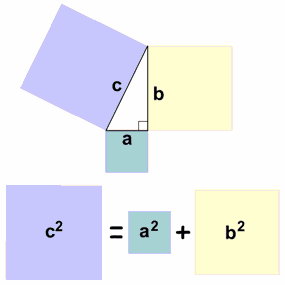Pythagoras was a famous Greek philosopher and Great Mathematician in 495BC. Pythagoras has been discovered a theorem in Geometry called after his name as”Pythagoras Theorem”. In his theorem he established the relation ship between the Hypotenuse and the other two sides adjacent to the right angle. Though this theorem now bears his name it was previously formulated and used by Indians and the Babylonians.
According to this theorem The Square of the hypotenuse is equal to the sum of the squares of the other to sides. In this formula finding any one of the unknown quantity is a long process of taking square root. It is a cumbersome time consuming process.
But before his period, The Tamil people in India followed a easy way to calculate the lengths of the sides with out taking Square roots. The Tamil Poet Bothaiyanar mentioned this method in his Tamil verses. I have given you the details with the pictures please compare them and point out which is the easiest way to find the length.
According to the Bothaiyanar to find the length of the hypotenuse, measure any one of the sided and subtract one eighth of its length from it and add it with half of the other side you will get the length of the hypotenuse.

It is very simple isn’t it ancient Tamilians find the height and slope of the hills by this way only
And this is your turn, please register which is the easy method to calculate?

1.Bothaiyanar is easier because it uses only the basic Math and no squares are involved. However, I saw a glitch in the instructions. If we follow the basic instructions above and I would choose the other side b that measures 6 to be diminished by 1/8 of its length this would give:

c = (b - b/8) + (a/2)
= (6 - 0.75) + (8/2)
= 5.25 + 4
= 9.25

Bothaiyanar should have indicated to use the longer side to be subtracted by 1/8 of its length and the shorter side to be divided by two.

2.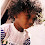I agree what you said is correct.

3.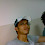well.
maybe Bothainayar method is easier. But it's all in the past. They don't have calculator back then. So adding is an easier method.
The technology has been developing rapidly and it seems that most of people tend to use Pythagoras method nowadays. Either way, big hand for both of them for being able to think the unthinkable. (^^)

Cheers from me. sUB™

4.8c=7a+4b is much easier...we can easily find either a or b or c provided we are good in multiplication and addition.. This equation would be easier to solve using a calculator too.. good post friend...

5.Who is Bothaiyanar, how to know about him ?

M S VINCENT

6.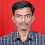8c=7a+4b is nothing but the simplification of Bothaiyanar c=a-a/8+b/2

7.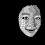its interesting!!!

But sadly it will give the exact solution provided the ratio of a:b is 4:3 or 12:5. However its giving the good approximation between these two ratios. But if we choose the a:b ratio more than 3, the error is increasing quadratically. With some modification we can rectify this drawback. I hope some one will come up with neo-bothaiyanar theorem :)

anbudan
Prakash

8.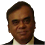@Prakash //But if we choose the a:b ratio more than 3, the error is increasing quadratically.//

In the Bothaiyanar equation c=(7/8)a+(1/2)b, consider when b->0 for finite a, then c-->(7/8)a whereas Pythagorean correct hypotenuse -->a, so the error being (1/8)a is 12.5%.

Similarly at the other end when a->0 error by Bothaiyanar is a muc larger 50%

If you take the error as C-c wherein C being estimate by Pythegorus theorem and c from Bothaiyanar equation then the error as a percentage of the correct value C being 100{1-(c/C)} can be plotted against a parameter x wherein the ratio x = (a/b), by the following equation :

100{1-(c/C)} = 100{1 - (1/8)(7x+4)/[(x^2+1)^0.5] }

Have a look at my plot here:

For large x (i.e., when b tends to 0 or a tends to infinity), the asymptotic limit of the error as percent of correct value is 12.5% and that is actually the maximum bound on error for x > 1 (i.e. a>b)

Bothaiyanar's Tamil verse actually distingushes a and b by detailing to take 7/8 portion of one "Odum NeeLam (ஓடும் நீளம்) and take half of a "KunRam (குன்றம்). Obviously from the error plot we could conclude he could have only meant ஓடும் நீளம் to be the larger of the two sides and the குன்றம் to be the shorter.

I think a maximum error of 12.5% in the ancient period of no claculating devices would have been very much accepatable for preliminary estimations such as designing of a service ramp for a tall tower construction.

9.The URL for my plot which i wrote above inadvertently includes a comma at the end. The following is the corrected URL:

10.